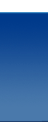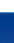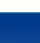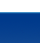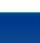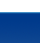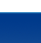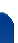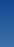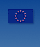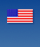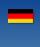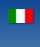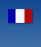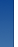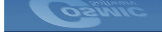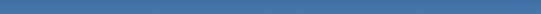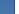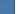Cosmic Software Frequently Asked Questions How do I access the CCR (Code Condition Register) from my C program? In general, you can create a simple macro with inline assembly to access the Condition Code Register or any other special instructions. The following examples demonstrate how to retrieve the CCR using various processors. The CCR is not memory mapped so it can only be obtained using special instructions and cannot be modified by a simple read and write. The following macros define a GET_CCR and PUT_CCR macros that may be used in an application. Examples ```// HC05 Compiler Definitions #define GET_CCR (unsigned char)(_asm("tpa") ) #define PUT_CCR(X) (_asm("tap\n", ((unsigned char)X )) ) // HC08 Compiler Definitions #define GET_CCR (unsigned char)(_asm("tpa") ) #define PUT_CCR(X) (_asm("tap\n", ((unsigned char)X )) ) // HC11 Compiler Definitions #define GET_CCR (unsigned char)(_asm("tpa \n tab \n") ) #define PUT_CCR(X) (_asm("tba \n tap\n", ((unsigned char)X )) ) // HC12 Compiler Definitions #define GET_CCR (unsigned char)(_asm("tfr ccr, b") ) #define PUT_CCR(X) (_asm("tfr b,ccr", ((unsigned char)X )) ) // Usage for 8 bit CCR #define BIT5 (unsigned char) 0x10 unsigned char var; var = GET_CCR; OR to test certain bits if ( (unsigned char)(GET_CCR & BIT4)) to write the CCR PUT_CCR(var); // HC16 Compiler Definition #define GET_CCR (unsigned int)_asm("tpd") #define PUT_CCR(X) (_asm("tpd \n", ((unsigned int)X )) ) // 68300 Compiler Definition #define GET_CCR (unsigned int) _asm("move ccr,d7") #define PUT_CCR(X) (_asm("move d7,ccr ", ((unsigned int)X )) ) // Usage for 16 bit CCR unsigned int var; var = GET_CCR; OR to test certain bits if ( (GET_CCR & BIT5) to write the CCR PUT_CCR(var); ```